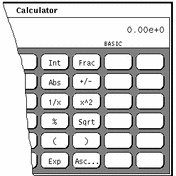OpenWindows User's Guide

## Number Manipulation Functions

The Calculator has 12 number manipulation functions, as shown in Figure 8-7.

##### Figure 8-7 Number Manipulation Functions• Int returns the integer portion of the currently displayed value.

• Frac returns the fractional portion of the currently displayed value.

• Abs returns the absolute value of the currently displayed value.

• +/- changes the arithmetic sign of the currently displayed value or of an exponent being entered with the Exp key.

• 1/x returns the value of 1 divided by the currently displayed value.

• x^2 returns the square of the currently displayed value.

• % calculates the percentage of the currently displayed value specified by the next value entered. For example, to find 75% of 40, enter 40, click SELECT on %, enter 75, then click SELECT on =. The Calculator returns the result, 30.

• Sqrt returns the square root of the currently displayed value.

• Parentheses give a calculation arithmetic precedence. All calculations within parentheses are calculated first. Parentheses can be nested. The result of an operation containing parentheses is not performed until the last parenthesis is matched.

• Exp starts exponential input. Any number entered after you choose Exp is taken to be the exponent portion of this number. The numbers entered before the Exp key are taken to be the mantissa. If no numerical input has occurred when the Exp key is entered, a mantissa of 1.0 is assumed. You can use the +/- key after entering the exponent to change its arithmetic sign.

• Asc displays the ASCII value of a character in the appropriate numeric base. Clicking on the Asc key displays a small pop-up window. Type the character that you want an ASCII equivalent for in the Character text field of the Get ASCII window, and then click SELECT on the ASCII button. The ASCII equivalent becomes the current value in the Calculator base window.